XLua 与 ILRuntime 性能测试https://github.com/Ourpalm/ILRuntime

https://github.com/Tencent/xLua

Xlua 生成了 Vector3_Wrap
.Net 3.5版本下

Test1 测试U3d内部值计算

ILRuntime:
[cc lang=”C#”]
public void Test1()
{
Stopwatch sw = new Stopwatch();
sw.Start();
for(int i = 0; i < 1000000; i++) { Vector3 a = new Vector3(1, 2, 3); Vector3 b = new Vector3(4, 5, 6); Vector3 c = a + b; } sw.Stop(); UnityEngine.Debug.Log("il test1:" + sw.ElapsedMilliseconds); } [/cc] Xlua: [cc lang="lua"] void LuaTest1() { System.Diagnostics.Stopwatch sw = new System.Diagnostics.Stopwatch(); sw.Start(); env.DoString(@" for i = 0, 1000000, 1 do local a = CS.UnityEngine.Vector3(1,2,3) local b = CS.UnityEngine.Vector3(4,5,6) local c = a + b end "); sw.Stop(); Debug.Log("lua test1:" + sw.ElapsedMilliseconds); } [/cc]

使用xlua 进行Unity3D 热更新-2

xlua的热修复原理实际上是在 C# 编译成中间语言的时候，进行代码的插入这部分用到了 Mono.Ceil 库来操作，当然还有其他很多的库也可以实现。 因为是在IL的部分插入，因此直接支持IL2CPP

[cc lang=”C++”]
public class InputTest{

void Start(){
Hello();
}
private void Hello(){
Debug.Log(“hello”);
Debug.Log(“666”):
}
}
[/cc]

[cc lang=”C++”]
string injectPath = @”./Library\ScriptAssemblies\Assembly-CSharp.dll”;
AssemblyDefinition assemblyDefinition = null;
[/cc]

[cc lang=”C++”]
foreach (Mono.Cecil.TypeDefinition item in assemblyDefinition.MainModule.Types) {

if(item.FullName == “InputTest”) {
foreach (MethodDefinition method in item.Methods) {

if (method.Name.Equals(“Hello”)) {
}
}
}
}
[/cc]

[cc lang=”C++”]
var ins = method.Body.Instructions.First();
var worker = method.Body.GetILProcessor();
var logRef = assemblyDefinition.MainModule.Import(typeof(Debug).GetMethod(“Log”, new Type[] { typeof(string) }));

worker.InsertBefore(ins, worker.Create(OpCodes.Ldstr, “Fuck Off”));
worker.InsertBefore(ins, worker.Create(OpCodes.Call, logRef));

worker.InsertBefore(ins, worker.Create(OpCodes.Ldstr, “Fuck On”));
worker.InsertBefore(ins, worker.Create(OpCodes.Call, logRef));

Type type = typeof(InjectTest);

if (null != type) {
MethodInfo subMethod = type.GetMethod(“SayFuck”);

if (null != subMethod) {
Debug.Log(“Find Method: ” + subMethod);

var sayRef = assemblyDefinition.MainModule.Import(subMethod);

worker.InsertBefore(ins, worker.Create(OpCodes.Call, sayRef));
}
}

var writerParameters = new WriterParameters { WriteSymbols = true };
assemblyDefinition.Write(injectPath, new WriterParameters());
[/cc]使用xlua 进行Unity3D 热更新

github地址 : xlua

[cc lang=”C++”]
void Start()
{
if(_hotfix)
else
}[/cc]

[cc lang=”C++”]
using System.Collections;
using System.Collections.Generic;
using UnityEngine;
using XLua;

[Hotfix]
public class FuckYouSelf : MonoBehaviour {

private int A;
private int B;

void Init() {

}
// Use this for initialization
void Start () {
Init();
}

int Add(int a, int b) {
return a – b;
}

// Update is called once per frame
void Update () {

}
}
[/cc]

[cc lang=”C++”]
using System;
using System.Collections;
using System.Collections.Generic;
using UnityEngine;
using XLua;

public class HotFix : MonoBehaviour {

public GameObject StartUp;

[Serializable]
struct VersionData {
public string Version;
public string FixUrl;
}

LuaEnv luaevn = new LuaEnv();
// Use this for initialization
void Awake () {

}

WWW versionData = new WWW(versionUrl);

yield return versionData;

if(null != versionData.error) {
Debug.LogError(versionData.error);
} else {
VersionData data = JsonUtility.FromJson(versionData.text);
}
}

IEnumerator LoadFix(string fixUrl, string version) {

//todo: check storage hotfix version

WWW fixData = new WWW(fixUrl);

yield return fixData;

if (null != fixData.error) {
Debug.LogError(fixData.error);
} else {
ApplyHotFix(fixData.text);
SaveToStorage(fixData.text, version);
}
}

void ApplyHotFix(string luastr) {
luaevn.DoString(luastr);

if(null != StartUp) {
StartUp.SetActive(true);
}
}

void SaveToStorage(string luastr, string version) {
//todo
}

private void OnDestroy() {

}
}
[/cc]

[cc lang=”lua”]
xlua.private_accessible(CS.FuckYouSelf)

xlua.hotfix(CS.FuckYouSelf, ‘Init’,
function(self)
self.A = 300
self.B = 300
end
)

function(self, a, b)
return a + b
end
)
[/cc]

lua部分的代码,就3段 第一段 xlua.private_accessible(CS.FuckYouSelf)

Unity3d 中的 HDR_BLOOM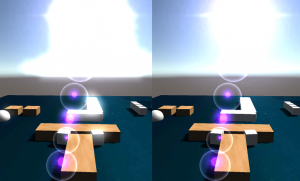float brightness = col.r * 0.299 + col.g * 0.587 + col.b * 0.114;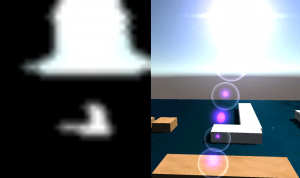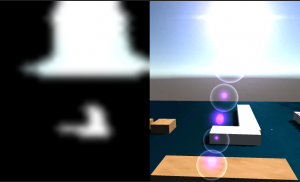{
Properties
{
_MainTex ("Texture", 2D) = "white" {}
_Brightness("BrightnessBias", float) = 0.1
}
{
Tags { "RenderType"="Opaque" }
LOD 100

Pass
{
CGPROGRAM
#pragma vertex vert
#pragma fragment frag
// make fog work
#pragma multi_compile_fog

#include "UnityCG.cginc"

struct appdata
{
float4 vertex : POSITION;
float2 uv : TEXCOORD0;
};

struct v2f
{
float2 uv : TEXCOORD0;
UNITY_FOG_COORDS(1)
float4 vertex : SV_POSITION;
};

sampler2D _MainTex;
uniform float _Brightness;
float4 _MainTex_ST;

v2f vert (appdata v)
{
v2f o;
o.vertex = mul(UNITY_MATRIX_MVP, v.vertex);
o.uv = TRANSFORM_TEX(v.uv, _MainTex);
UNITY_TRANSFER_FOG(o,o.vertex);
return o;
}

fixed4 frag (v2f i) : SV_Target
{
// sample the texture
fixed4 col = tex2D(_MainTex, i.uv);
float brightness = col.r * 0.299 + col.g * 0.587 + col.b * 0.114;

if (brightness < _Brightness) {
col.rgb = 0;
}

// apply fog
UNITY_APPLY_FOG(i.fogCoord, col);
return col;
}
ENDCG
}
}
}

2×2模糊

{
Properties
{
_MainTex ("Texture", 2D) = "white" {}
}
{
Tags { "RenderType"="Opaque" }
LOD 100

Pass
{
CGPROGRAM
#pragma vertex vert
#pragma fragment frag

#include "UnityCG.cginc"

struct appdata
{
float4 vertex : POSITION;
float2 uv : TEXCOORD0;
};

struct v2f
{
float2 uv : TEXCOORD0;
float4 leftUpUV : TEXCOORD1;
float4 rightDownUV : TEXCOORD2;
float4 vertex : SV_POSITION;
};

sampler2D _MainTex;
float4 _MainTex_ST;
float4 _MainTex_TexelSize;

v2f vert (appdata v)
{
v2f o;
o.vertex = mul(UNITY_MATRIX_MVP, v.vertex);

float2 uv = TRANSFORM_TEX(v.uv, _MainTex);
o.uv = uv;
o.leftUpUV = float4(uv.x - _MainTex_TexelSize.x, uv.y, uv.x, uv.y + _MainTex_TexelSize.y);
o.rightDownUV = float4(uv.x + _MainTex_TexelSize.x, uv.y, uv.x, uv.y - _MainTex_TexelSize.y);

return o;
}

fixed4 frag (v2f i) : SV_Target
{
// sample the texture
fixed4 col = tex2D(_MainTex, i.uv);

col += tex2D(_MainTex, i.leftUpUV.xy);
col += tex2D(_MainTex, i.leftUpUV.zw);
col += tex2D(_MainTex, i.rightDownUV.xy);
col += tex2D(_MainTex, i.rightDownUV.zw);

col /= 5;

return col;
}
ENDCG
}
}
}

using UnityEngine;
using System.Collections;

public class HDRBloomPostEffect : MonoBehaviour {
public float BrightnessBias = 1.0f;

private Material m_hdrBloomMat;
private Material m_filterMat;
private Material m_combineMat;

// Use this for initialization
void Start () {
}

float ComputeGaussianWeight(int posX, int posY, float sigma)
{
float v1 = 1.0f / (2.0f * Mathf.PI * sigma * sigma);
float v2 = -(posX * posX + posY * posY) / (2 * sigma * sigma);
v2 = Mathf.Exp(v2);

return v1 * v2;
}

void OnRenderImage(RenderTexture src, RenderTexture dst) {
RenderTexture rt16 = RenderTexture.GetTemporary(src.width / 16, src.height / 16);
RenderTexture rt8 = RenderTexture.GetTemporary(src.width / 8, src.height / 8);
RenderTexture rt4 = RenderTexture.GetTemporary(src.width / 4, src.height / 4);
RenderTexture rt2 = RenderTexture.GetTemporary(src.width / 2, src.height / 2);

m_hdrBloomMat.SetFloat("_Brightness", BrightnessBias);
Graphics.Blit(src, rt16, m_hdrBloomMat);

Graphics.Blit(rt16, rt8, m_filterMat);
Graphics.Blit(rt8, dst, m_filterMat);
Graphics.Blit(rt4, rt2, m_filterMat);

m_combineMat.SetTexture("_AnotherTex", src);
Graphics.Blit(rt2, dst, m_combineMat);

RenderTexture.ReleaseTemporary(rt16);
RenderTexture.ReleaseTemporary(rt8);
RenderTexture.ReleaseTemporary(rt4);
RenderTexture.ReleaseTemporary(rt2);
}
}

榨干GPU 使用顶点颜色信息来创建千千万的高性能植被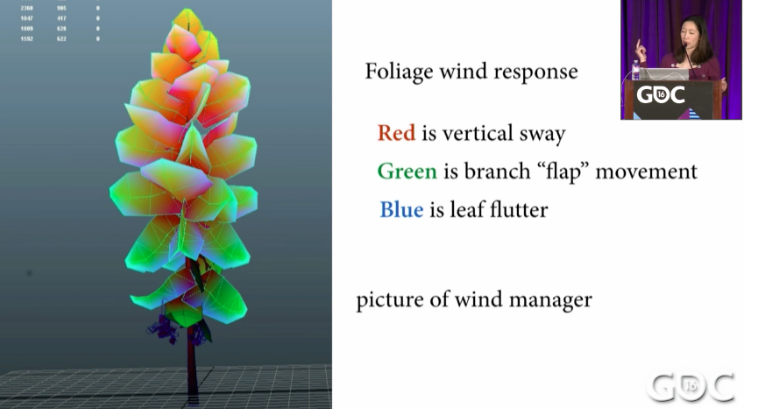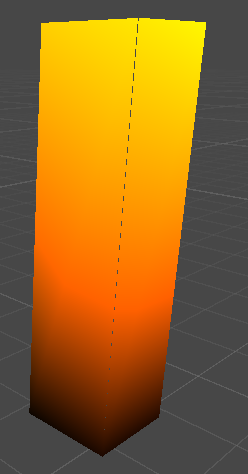v2f vert (appdata v)
{
v2f o;
float scale = cos(_Time.y * _Speed) * v.color.r * (v.color.g);float szControl = randValue > 0.5;
v.vertex.z += scale * szControl;
v.vertex.x += scale * (1.0 – szControl);

float4 worldPos = mul(_Object2World, v.vertex);

o.normal = normalize(mul(_Object2World, v.normal));
o.worldPos = worldPos;
o.pos = mul(UNITY_MATRIX_VP, worldPos);
o.uv = TRANSFORM_TEX(v.texcoord, _MainTex);

return o;
}

fixed4 frag (v2f i) : SV_Target
{
float3 lightDir = normalize(WorldSpaceLightDir(i.worldPos));
float3 viewDir = normalize(_WorldSpaceCameraPos – i.worldPos);
float3 halfDir = normalize(lightDir + viewDir);
float3 diffuse = tex2D(_MainTex, i.uv) * max(0, dot(i.normal, lightDir));
float3 specular = _LightColor0.rgb * pow(max(0, dot(i.normal, halfDir)), _Shiness);
float4 finalCol = float4(diffuse + specular + UNITY_LIGHTMODEL_AMBIENT, 1.0);

return finalCol;
}